# Exercise E7.4 Triangles NCERT Solutions Class 9

## Chapter 7 Ex.7.4 Question 1

Show that in a right angled triangle, the hypotenuse is the longest side.

### Solution

What is Known?

A right-angled triangle.

To prove:

Hypotenuse is the longest side.

Reasoning:

In a triangle if one angle is of $$90$$ degree then the other two angles have to be acute and we can use the fact that in any triangle, the side opposite to the larger (greater) angle is longer.

Steps: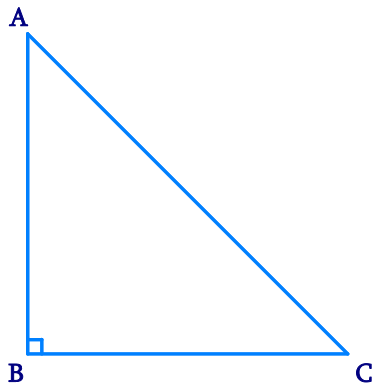Let us consider a right-angled triangle $$ABC$$, right-angled at $$B$$. In $$\Delta ABC$$,

\begin{align} & \angle A + \angle B + \angle C = 180^{\circ} \\&(\text{Angle sum property of a triangle})\\\\ & \angle A + 90^{\circ} + \angle C = 180^{\circ}\\ & \angle A + \angle C = 90^{\circ} \end{align}

Hence, the other two angles have to be acute (i.e., less than $$90^{\circ}$$). $$\angle B$$ is the largest angle in $$\Delta ABC$$.

$$\angle B \gt \angle A$$  and  $$\angle B \gt \angle C$$

$$AC \gt BC$$  and  $$AC \gt AB$$

[ In any triangle, the side opposite to the larger (greater) angle is longer.]

Therefore, $$AC$$ is the largest side in $$\Delta ABC$$.

However, $$AC$$ is the hypotenuse of $$\Delta ABC$$.

Therefore, hypotenuse is the longest side in a right-angled triangle.

## Chapter 7 Ex.7.4 Question 2

In the given figure sides $$AB$$ and $$AC$$ of $$\Delta ABC$$ are extended to points $$P$$ and $$Q$$ respectively. Also, $$\angle PBC \lt \angle QCB$$. Show that $$AC \gt \angle AB$$.

### Solution

What is Known?

$$\angle \text{PBC}<\angle \text{QCB}\text{.}$$

To prove:

$$\text{AC}>\text{AB}\text{.}$$

Reasoning:

By using linear pair, we can find inequality of interior angles and then we can use the fact that In any triangle, the side opposite to the larger (greater) angle is longer.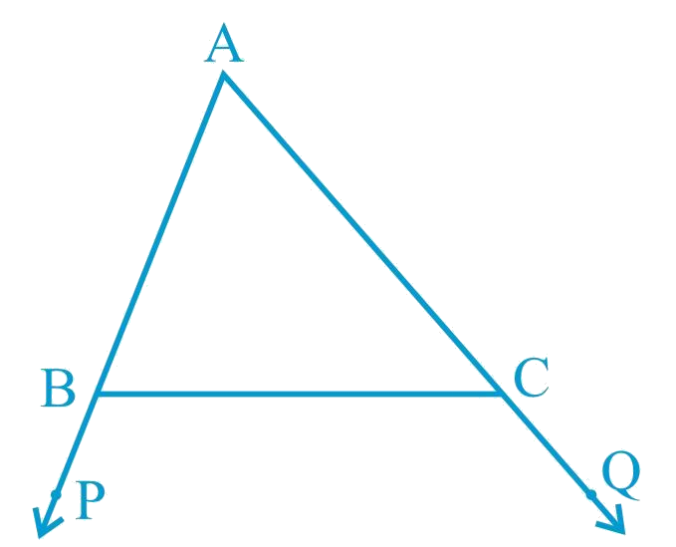Steps:

In the given figure,

\begin{align} &\angle ABC +\angle PBC=180^{\circ}\,\,\,\\&\text{( Linear pair)} \\\\& \angle ABC =180^{\circ} - \angle PBC\, \ldots (1) \\ \end{align}

Also,

\begin{align} &\angle ACB +\angle QCB=180^{\circ} \\ &\angle ACB =180^{\circ} - \angle QCB\, \ldots (2) \\ \end{align}

As $$\angle PBC \lt \angle QCB$$,

\begin{align} & 180^{\circ} - PBC \gt 180^{\circ} - \angle QCB\\ & \angle ABC \gt ACB\\&[\text{From Equations (1) and (2)}]\\\\ & AC \gt AB \\&\left (\begin{array}\ \text{side opposite to the}\\\text{ larger angle is larger.}\end{array} \right)\end{align}

Hence proved, $$AC \gt AB$$

## Chapter 7 Ex.7.4 Question 3

In the given figure, $$\angle B \lt \angle A$$ and

$$\angle C \lt \angle D$$. Show that $$AD \lt BC$$.

### Solution

What is Known?

$\angle \text{B}<\angle \text{A and }\angle \text{C}<\angle \text{D}$

To prove:

$\text{AD}<\text{BC}$

Reasoning:

We can use the fact that In any triangle, the side opposite to the larger (greater) angle is longer We can add both the triangles result to get the required result.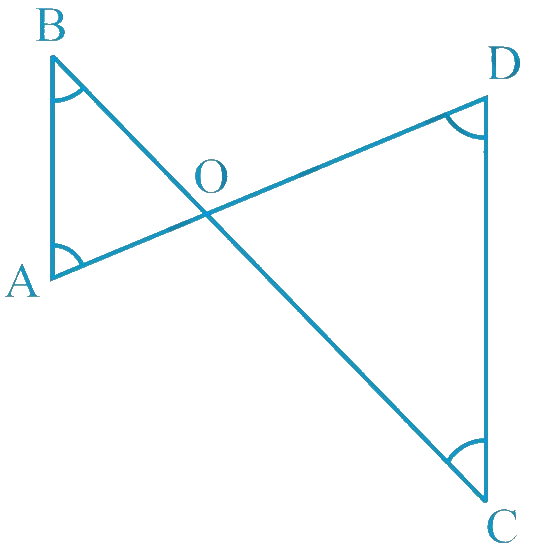Steps:

In $$\Delta AOB$$,

\begin{align}&\angle B \lt \angle A\\ &AO \lt BO\\& \left(\begin{array}{}\text{Side opposite to the smaller}\\ \text{ angle is smaller} \ldots (1)\end{array}\right)\end{align}

In $$\Delta COD$$,

\begin{align}&\angle C \lt \angle D\\ &OD \lt OC\\& \left(\begin{array}{}\text{Side opposite to the smaller}\\ \text{ angle is smaller} \ldots (2)\end{array}\right)\end{align}

On adding Equations ($$1$$) and ($$2$$), we obtain

\begin{align} & AO + OD \lt BO + OC \\ & AD \lt BC, \text{proved}\end{align}

## Chapter 7 Ex.7.4 Question 4

$$AB$$ and $$CD$$ are respectively the smallest and longest sides of a quadrilateral $$ABCD$$ (see the given figure). Show that $$\angle A \gt \angle C$$ and $$\angle B \gt \angle D$$.

### Solution

What is Known?

$$AB$$ and $$CD$$ are respectively the smallest and longest sides.

To prove:

$\angle \text{A}>\angle \text{C and }\angle \text{B}>\angle \text{D}$

Reasoning:

First of all we can join vertex A to C then triangles $$ABC$$ and $$ADC$$ will be formed now we can use the fact that In any triangle, the side opposite to the larger (greater) angle is longer We can add both the triangles result to get the first required result, Similarly we can join vertex B and D and use the fact to get the other required result.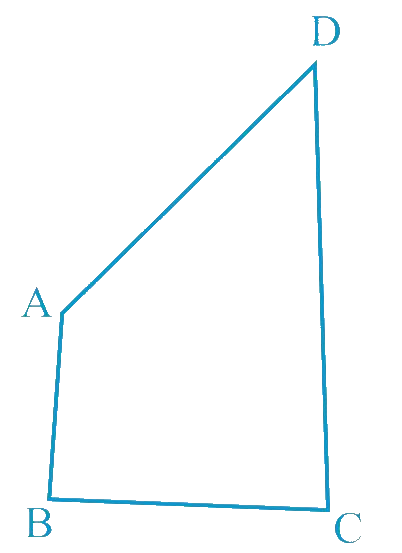Steps:

Let us join $$AC$$.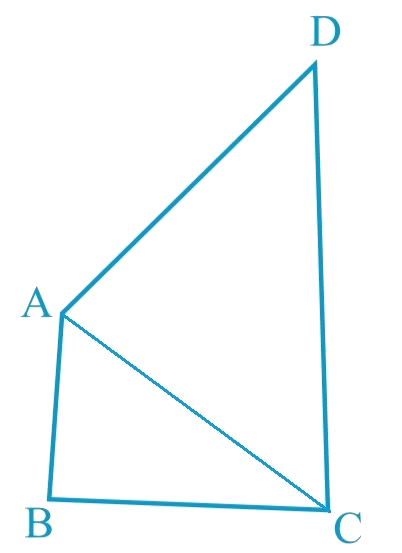In $$\Delta ABC$$,

\begin{align}&AB>BC\\&\left( \begin{array} & \text{AB is the largest side } \\ \text{of quadrilateral}\,\,ABCD \\ \end{array} \right)\\\\&\angle2>\angle1\\&\left( \begin{array} & \text{Angle opposite to the } \\ \text{smaller side is smaller} \\ \end{array} \right)...\left( \text{1} \right)\end{align}

In $$\Delta ADC$$,

\begin{align}&AB>BC\\&\left( \begin{array} & \text{CD is the largest side } \\ \text{of quadrilateral}\,\,ABCD \\ \end{array} \right)\\\\&\angle4>\angle3\\&\left( \begin{array} & \text{Angle opposite to the } \\ \text{smaller side is smaller} \\ \end{array} \right)...\left( \text{2} \right)\end{align}

On adding Equations ($$1$$) and ($$2$$), we obtain

\begin{align}& \angle 2 + \angle 4 \lt \angle 1 + \angle 3\\ & \angle C \lt \angle A \\ & \angle A \gt \angle C \end{align}

Let us join $$BD,$$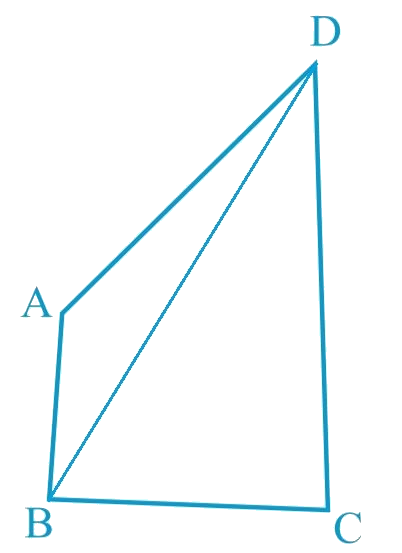In $$\Delta ABD$$,

\begin{align}&AB>AD\\&\left( \begin{array} & \text{AB is the largest side } \\ \text{of quadrilateral}\,\,ABCD \\ \end{array} \right)\\\\&\angle8>\angle5\\&\left( \begin{array} & \text{Angle opposite to the } \\ \text{smaller side is smaller} \\ \end{array} \right)...\left( \text{3} \right)\end{align}

In $$\Delta BDC$$,

\begin{align}&BC>CD\\&\left( \begin{array} & \text{CD is the largest side } \\ \text{of quadrilateral}\,\,ABCD \\ \end{array} \right)\\\\&\angle7>\angle6\\&\left( \begin{array} & \text{Angle opposite to the } \\ \text{smaller side is smaller} \\ \end{array} \right)...\left( \text{4} \right)\end{align}

On adding Equations ($$3$$) and ($$4$$), we obtain

\begin{align}& \angle 8 + \angle 7 \lt \angle 5 + \angle 6\\ & \angle D \lt \angle B \\ & \angle B \gt \angle D \text{ (Hence, proved)}\end{align}

## Chapter 7 Ex.7.4 Question 5

In the given figure, $$PR \gt PQ$$ and $$PS$$

bisects $$\angle QPR$$. Prove that

$$\angle PSR \gt \angle PSQ$$.

### Solution

What is Known?

$$\text{PR PQ}$$ and PS bisects $$\angle \text{QPR}$$

To prove:

$\angle \text{PSR }>\angle \text{PSQ}\text{.}$

Reasoning:

We can use exterior angle sum property to find the required inequality.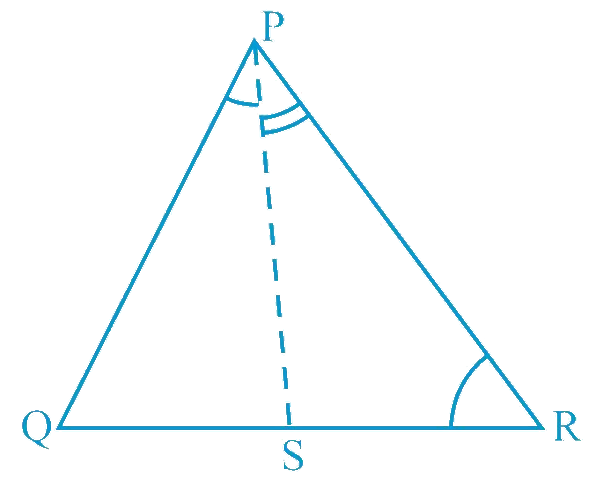Steps:

As $$PR \gt PQ$$,

\begin{align} & \angle PQR \gt \angle PRQ\\&\left(\begin{array}{} \text {Angle opposite to larger}\\\text{ side is larger}) \ldots (1)\end{array}\right) \\\\ & PS \text { is the bisector of } \angle QPR \\ & \angle QPS = \angle RPS \ldots (2) \\\\ & \angle PSR \text{ is the exterior angle of } PQS \\ & \angle PSR = \angle PQR + \angle QPS \ldots (3)\\\\ & \angle PSQ \text{ is the exterior angle of } PRS \\ & \angle PSQ = \angle PRQ + \angle RPS \ldots (4)\\ \end{align}

Adding Equations ($$1$$) and ($$2$$), we obtain

\begin{align} &\angle PQR + \angle QPS \gt \angle PRQ + \angle RPS \\ & \angle PSR \gt \angle PSQ \\& \begin{bmatrix}\text{Using the values of}\\ \text{Equations (2),(3) and (4)}\end{bmatrix}\end{align}

## Chapter 7 Ex.7.4 Question 6

Show that of all line segments drawn from a given point not on it, the perpendicular line segment is the shortest.

### Solution

What is Known?

Line segments drawn from a given point is not on line.

To prove:

The perpendicular line segment is the shortest.

Reasoning:

We know that in a triangle if one angle is $$90$$ degree then other have to be acute.

Steps: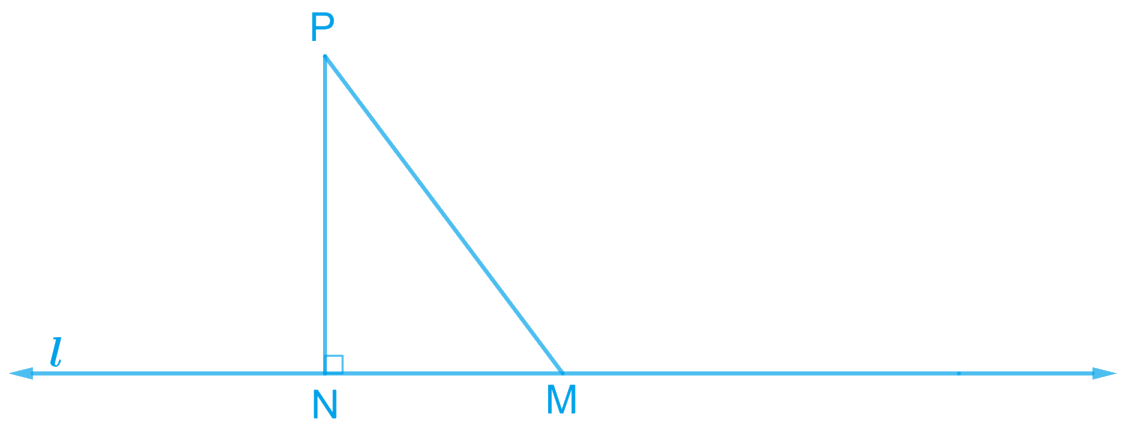Let us take a line $$l$$ and from point $$P$$ (i.e., not online $$l$$), draw two line segments $$PN$$ and $$PM$$. Let $$PN$$ be perpendicular to line $$l$$ and $$PM$$ is drawn at some other angle.

In $$\Delta PNM$$,

\begin{align} & \angle N = 90 ^ { \circ } \\ & \angle P + \angle N + \angle M = 180 ^ { \circ }\\& \left( \begin{array} & \text{Angle sum property } \\ \text{of a triangle} \\ \end{array} \right)\\ \\ & \angle P + \angle M = 90 ^ { \circ } \end{align}

Clearly, $$\angle M$$ is an acute angle.

\begin{align} & \angle M \lt \angle N \\ & PN \lt PM \\&\left(\begin{array}{} \text {side opposite to the} \\\text{smaller angle is smaller}\end{array}\right) \end{align}

Similarly, by drawing different line segments from $$P$$ to $$l$$, it can be proved that $$PN$$ is smaller in comparison to them. Therefore, it can be observed that of all line segments drawn from a given point not on it, the perpendicular line segment is the shortest.

Instant doubt clearing with Cuemath Advanced Math Program